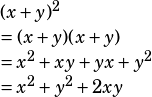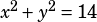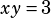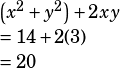If x^2 + y^2 = 14 and xy = 3, which of the following is equal to (x + y)^2?

Could you go step by step?

The way to get this one is to recognize that those are all parts of the same puzzle, which you do if you know your binomial squares pretty well. Here’s what I mean.

FOIL out thepart:From that, you should see that you have values for all those parts! The question tells you thatand that. So you can plug those values into the expression you found is equivalent to.Does that help?